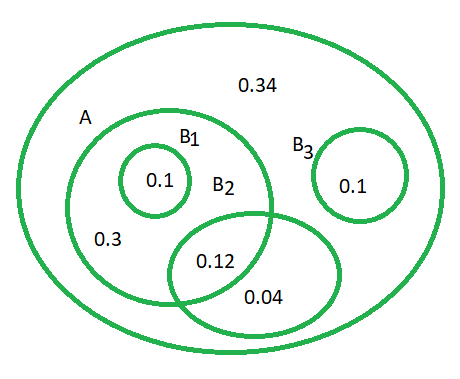# Molar Concentration Formula

• Last Updated : 08 Mar, 2022

The most convenient form of describing the concentration of a solute in a particular solution is molar concentration. The number of moles of the solute dissolved per litre of solution is known as molarity. As a result, M = moles per liter. The amount in moles of the solution, which is the molar concentration, will be determined by all mole computations.A mole ratio between the acid and the base is always the result of a balanced chemical equation. As a result, the number of moles in the base increases. By dividing the number of moles by the volume of the solution, we may get the molar concentration. All moles values are used to calculate the molar concentration, which is the volume of moles in the solution.

Formula

Molar concentration of a solution is calculated as the ratio of the amount in moles to the volume of the given solution. Its formula is given as follows:

Molar Concentration = Amount in moles/Volume of the solution

### Sample Problems

Question 1. If 16.35 mL of an AgNO3 solution reacts with 0.0003017 kg of KIO3, find its concentration.

Solution:

AgNO3 + KIO3 = AgIO3 + KNO3

Number of moles in KIO3 = Mass/Molar massHence, Number of moles in AgNO3 = 0.00141 mol

Now, Concentration of AgNO3 = Moles/Volume

Concentration of AgNO3 = (0.00141)/(0.01635)

= 0.08624 M

Question 2. What is the molarity formula used for?

Solution:

The concentration formula in chemistry is utilized in a variety of branches of study. This formula is frequently used in advanced science courses to assist students comprehend the kinetics of chemical reactions. In fact, molarity may be used to evaluate the influence of thermal expansion in fluids. This formula can be used to compute the amounts of reacting substances or the quantity necessary for a reaction to produce a calculated output.

Question 3. Find the molarity of 4 g of caustic soda, if it was in 200 ml of solution.

Solution:

Mass = 4 g

Volume = 200 ml

Molar mass of caustic soda = 23 + 16 + 1 g

= 40 g

Molarity = Mass/Molar Mass

= 4g/40g

= 0.1 g

Question 4. 15 g sodium sulphate was used to make a solution. The solution has a volume of 125 ml. Find the molarity of sodium sulphate solution supplied.

Solution:

Molar mass of sodium sulphate = 23 × 2 + 32 + 16 × 4 = 142 g

Number of moles in sodium sulphate = 15/142 = 0.106

Volume of the solution = V = 0.125l

Thus, molarity = n/V

= 0.106/0.125

= 0.85 M

Question 5. Determine the molar concentration of sodium hydroxide for its reaction with hydrochloric acid.

Solution:

HCl + NaOH → NaCl + H2O

Number of moles in HCl = Number of moles in NaOH = 8.75 × 10-3 mol.

The amount of NaOH present in moles is

Volume of solution = 25 × 10-3dm3

M = 8.75 × 10−3/25.0 × 10-3

= 0.350 mol dm-3

My Personal Notes arrow_drop_up